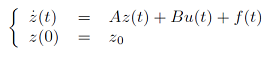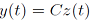##### Feedback Controls for Finite Time or Asymptotic Compensation in Lumped Disturbed Systems

L. Afifi, K. Lasri, M. Joundi, N. Amimi

Journal of Advances in Mathematics and Computer Science, Page 168-180
DOI: 10.9734/BJMCS/2015/14872

In this paper, we study the possibility of finite time or asymptotic compensation of disturbances f,
for a class of linear lumped systemsaugmented with the output equationusing directly feedback controls u = K f, where K is a linear operator. We give appropriate
definitions and characterization results of this notion, called K-remediability. We also examine the
relationship with the remediability as studied in previous works. Illustrative examples are presented
and various situations are considered. The relation u = K f includes the usual form u = K yf ,
where yf is the term corresponding, in the expression of the observation y, to the disturbance f. In
the linear case, yf may be deduced easily from the observation y, even if f is not known.

##### A New Approach to (∈, ∈ ∨q)-Fuzzy Subgroup Theory via Soft Sets

Yıldıray Çelik

Journal of Advances in Mathematics and Computer Science, Page 181-190
DOI: 10.9734/BJMCS/2015/15518

In this paper, we give the notion of (∈, ∈ ∨q)-fuzzy soft group and introduce its some properties and structural characteristics. Moreover, the definition of (∈, ∈ ∨q)-fuzzy normal soft group is given and some of its basic properties are studied. Also, the theorem regarding of homomorphic image and homomorphic pre-image of a (∈, ∈ ∨q)-fuzzy soft group under a fuzzy soft function is given.

##### A Class of Riemannian Manifolds with Special Form of Curvature Tensor

Musa A. A. Jawarneh

Journal of Advances in Mathematics and Computer Science, Page 191-196
DOI: 10.9734/BJMCS/2015/15366

Riemannian curvature is determined by the Ricci curvature by a special formula to introduce a special class of Riemannian manifolds which called Jawarneh manifold. Some geometric properties of Jawarneh manifold have been derived and a non-trivial example is obtained to prove the existence.

##### LQ Optimal Control-Based Variable Gain Controllers for Linear Systems with Structured Uncertainties and Its Performance Analysis

Hidetoshi Oya, Yuhei Uehara

Journal of Advances in Mathematics and Computer Science, Page 197-208
DOI: 10.9734/BJMCS/2015/14446

This paper proposes a variable gain controller for a linear system with structured uncertainties. The proposed variable gain controller is based on LQ optimal control for the nominal system and consists of the optimal feedback gain and a time-varying adjustable parameter which is designed so as to reduce the effect of uncertainties. The proposed LQ optimal control-based variable gain controller can achieve good transient performance which is close to LQ optimal control for the nominal system and adjust the magnitude of the control input. In this paper, we show sufficient conditions for the existence of the proposed variable gain robust controller for the uncertain linear system. Finally, an illustrative example is included.

##### Optimal Homotopy Analysis Methods for Solving the Linear and Nonlinear Fokker-Planck Equationsa

Shreekant P. Pathak, Twinkle Singh

Journal of Advances in Mathematics and Computer Science, Page 209-217
DOI: 10.9734/BJMCS/2015/15230

In this paper, linear and nonlinear Fokker-Planck equations are solved by approximate method, namely Optimal homotopy analysis method (OHAM). The Optimal homotopy analysis method is a combination of the homotopy analysis method and optimizing the convergence control parameter by minimizing the square residual error. The mathematical calculation and graphics have been obtained. The results have been obtained by OHAM are matches with the exact results and other approximate method like, Adomian decomposition method (ADM), Variation iteration method (VIM) and Homotopy perturbation method (HPM).

##### Robust Electronic Communication Scheme in Spatial Domain

Aqsa Rashid

Journal of Advances in Mathematics and Computer Science, Page 218-228
DOI: 10.9734/BJMCS/2015/15228

With the growing tempo of not permitted right to use and assail, protection of private facts is of extreme value. These concerns results in Steganography. Simple least significant bit matching and least significant bit substitution techniques and some other methods have an advantage that after hiding the message, the distortion and statistical change in image is least. The purposed method uses the advantages of these methods and removes the negative aspect connected with them. Theoretically and practically, it is very difficult to break the proposed method. It is implemented for both the grayscale and color images.

##### Development of Okike’s Merged Irregular Transposition Cipher and Its Level Error

Okike Benjamin, E. J. D Garba

Journal of Advances in Mathematics and Computer Science, Page 229-240
DOI: 10.9734/BJMCS/2015/14869

Okike’s Merged Irregular Transposition Cipher is a new technique for enciphering information along the superhighway. In this method, a message to be transmitted along the superhighway is first split into parts and each part is encrypted separately before transmission occurs. The positions of the split encrypted messages may be swapped. This work deployed a message with approximately 58 characters to study the behavior of the error level as the message is split from 2 through 10. It was observed that as the number of split increases, the error level decreases. Similarly, the optimal split number occurs when the integer value of the error begins to repeat as is seen when the split number equals 8.